# implementation of queue

#### aspirea5745

Joined Apr 17, 2019
81
Hi there!

Let's assume there is a real life example of queue 2 4 6 7 (FIFO)

Queue = 2 4 6 7
Queue = 7 2 4 6
Queue = 6 7 2 4
Queue = 4 6 7 2
Queue = 2 4 6 7

I am trying to understand the process of queue in programming language.

I have tried this for, I think queue will be implement in programming like this. Does it make any sense to implement queue ?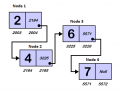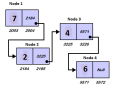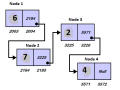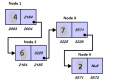#### Papabravo

Joined Feb 24, 2006
13,973
You have not described a queue. What you are describing is a cycle, where you remove an element from one end and put it on the other end. In a FIFO queue you always add elements at one using a "put" pointer. You remove them from the other end using a "get" pointer. In an EMPTY queue the "put" pointer is equal to the "get" pointer. Once you get an element, it is done with that queue.

#### aspirea5745

Joined Apr 17, 2019
81
You have not described a queue. What you are describing is a cycle, where you remove an element from one end and put it on the other end.
Is it FIFO queue ?
Queue = 2 4 6 7
Queue = 7 2 4 6
Queue = 6 7 2 4
Queue = 4 6 7 2
Queue = 2 4 6 7

In a FIFO queue you always add elements at one using a "put" pointer. You remove them from the other end using a "get" pointer. In an EMPTY queue the "put" pointer is equal to the "get" pointer. Once you get an element, it is done with that queue.
okay we have to pointer that can store data and address of next node. I do not understand how they will work to make queue ?

#### Papabravo

Joined Feb 24, 2006
13,973
Is it FIFO queue ?
Queue = 2 4 6 7
Queue = 7 2 4 6
Queue = 6 7 2 4
Queue = 4 6 7 2
Queue = 2 4 6 7

okay we have to pointer that can store data and address of next node. I do not understand how they will work to make queue ?
No you are showing a cylclic structure that always has 4 items in it. that is not how a queue works.

Would it help to imagine the queue shared by two processes. Process 1 adds items to the queue if it is not full. Process 2 takes items from the other end of the queue if it is not empty. Items in the queue effectively move from one end to the other AND process 2 takes and removes the items in the same order that process 1 put them in.

•aspirea5745

#### aspirea5745

Joined Apr 17, 2019
81

#### Papabravo

Joined Feb 24, 2006
13,973
alright let's try to make it on paper

View attachment 194850
There is one pointer that store data 2 and address of next node that is 2184.

What happens next ? should i create second node and first node and second should be connect with together ?
This picture would describe a queue with one element if the pointer were "Null". Since it points to 2184, the queue actually has two elements. Node 1 is the "head" of the queue. Node 2 @ 2184 would be the "tail" of the queue. A new item would be added to the queue by having Node 2 point to it. Items are taken from the "head", but they are added to the tail. With this structure you can only "walk" the queue in one direction: from "head" to "tail". You cannot walk it in the reverse direction.

•aspirea5745

#### aspirea5745

Joined Apr 17, 2019
81
This picture would describe a queue with one element if the pointer were "Null". Since it points to 2184, the queue actually has two elements. Node 1 is the "head" of the queue. Node 2 @ 2184 would be the "tail" of the queue. A new item would be added to the queue by having Node 2 point to it. Items are taken from the "head", but they are added to the tail. With this structure you can only "walk" the queue in one direction: from "head" to "tail". You cannot walk it in the reverse direction.
Is it a queue for two elements ?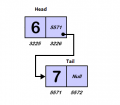#### Papabravo

Joined Feb 24, 2006
13,973
Is it a queue for two elements ?
View attachment 194867
Yes, that is a queue with two elements. To add a 3rd element you would update the pointer @ 5572 to point to it, and set it's pointer to Null. To take an item from the queue, you would use the head pointer to access it, then set the contents of the head pointer to 5571 so it points at the node with "7" in the data field. I think you have the idea.

•aspirea5745

#### aspirea5745

Joined Apr 17, 2019
81
Yes, that is a queue with two elements. To add a 3rd element you would update the pointer @ 5572 to point to it, and set it's pointer to Null. To take an item from the queue, you would use the head pointer to access it, then set the contents of the head pointer to 5571 so it points at the node with "7" in the data field. I think you have the idea.
@Papabravo Ok, let's try to convert thoughts into program

C:
#include<stdio.h>
#include <stdlib.h>
struct Queue
{
int Data;
struct Queue *next;
};
int main()
{
struct Queue *tail = NULL;
head = (struct Queue*) malloc(sizeof(struct Queue));
return 0;
}
This is my start to make queue. What do i have to do to make queue ?

#### Papabravo

Joined Feb 24, 2006
13,973
@Papabravo Ok, let's try to convert thoughts into program

C:
#include<stdio.h>
#include <stdlib.h>
struct Queue
{
int Data;
struct Queue *next;
};
int main()
{
struct Queue *tail = NULL;
head = (struct Queue*) malloc(sizeof(struct Queue));
return 0;
}
This is my start to make queue. What do i have to do to make queue ?
I suggest three functions of which you have written the first one which is to create an empty queue with no elements.
The second function takes an argument which is the data and adds that to the queue. It should be added to the tail, not the head. After updating the tail pointer to point to the new element, you check the head pointer to see if it is NULL. If it is you make head = tail.
The third function gets an item from the head an removes it from the queue. You should be able to tell from the return value if the queue is empty or not. You could also implement a function to test a queue for being empty and not calling the third function if it is.

•aspirea5745 and djsfantasi

#### aspirea5745

Joined Apr 17, 2019
81
I suggest three functions of which you have written the first one which is to create an empty queue with no elements.
Making the first function empty queue with no elements.
It will look like
C:
#include<stdio.h>
#include <stdlib.h>
struct Queue
{
int Data;
struct Queue *next;
};

void empty_queue()
{
struct Queue *tail = NULL;
head = (struct Queue*) malloc(sizeof(struct Queue));
}
int main()
{
return 0;
}
If it is correct i will try to make second function

#### Papabravo

Joined Feb 24, 2006
13,973
"head" and "tail" don't get valid values until the first item is placed in the queue.

#### djsfantasi

Joined Apr 11, 2010
6,532
Making the first function empty queue with no elements.
It will look like
C:
#include<stdio.h>
#include <stdlib.h>
struct Queue
{
int Data;
struct Queue *next;
};

void empty_queue()
{
struct Queue *tail = NULL;
head = (struct Queue*) malloc(sizeof(struct Queue));
}
int main()
{
return 0;
}
If it is correct i will try to make second function
What data do you expect to find in your “queue” structure? A pointer to the beginning? A pointer to the end? Any data at all?

I expected something like the following:
Code:
 struct QUEUE {
Data = NULL;
*Next = Null;
}
For a queue, you don’t need a tail.

Last edited:
•skyr6546

#### BobaMosfet

Joined Jul 1, 2009
1,124
Hi there!

Let's assume there is a real life example of queue 2 4 6 7 (FIFO)

Queue = 2 4 6 7
Queue = 7 2 4 6
Queue = 6 7 2 4
Queue = 4 6 7 2
Queue = 2 4 6 7

I am trying to understand the process of queue in programming language.

I have tried this for, I think queue will be implement in programming like this. Does it make any sense to implement queue ?

View attachment 194832

View attachment 194834

View attachment 194835

View attachment 194836
No, that is not a queue. A queue is a linked list of structures that ultimately point back to itself- a never-ending circle.

If a queue has 4 element (A, B, C, D), then A -> B, B -> C, C -> D, and D -> A.

That's a queue.

The way you manage a queue is by having two pointers, one for head, and one for tail. Both start out point at A. As A is loaded, head increments to B. Now the tail is A, and the head is B. When head increments again, B becomes C, while tail is still A. Only when some other thing pulls data out of A, does it pull the data from A and then increment tail to B. The idea is that you pull data out (from the tail) faster than the head is filled, that way, the head never runs into the tail. You can achieve this by making the tail eater faster, and/ or by ensuring your queue has enough elements in it, that it isn't likely to be overrrun.

A typical structure is this:

Code:
typedef struct qElemL
{
qElemL  *next;
int       data;                           // This can be any other type of data the queue entry needs to hold
}qElem,*qElemeP;

qElemP   tailQP;

// malloc a qElem for each element.  Remember the first one and make headQP and tailQP = to the first element.  As each new q element is allocated,  make the current element's next field point to it, and then make the current element the new one.  Until finally you have your queue allocated and the final q element next points back to address of the first element.
Remember to properly deallocate your queue when finished; don't just bail.

•aspirea5745

#### BobaMosfet

Joined Jul 1, 2009
1,124
I expected something like the following:
Code:
void empty_queue()
{
struct Queue *tail = NULL;
}

Do NOT use 'struct queue...' blah blah blah. That is bad programming. Anybody who taught you to preface a structure with 'struct' every time you use it is because they weren't bright enough themselves to understand what TYPEDEF is for. ALWAYS define your types. Then you can just use them without this amateurish 'struct' crap everywhere.

#### Papabravo

Joined Feb 24, 2006
13,973
A queue has both a head and a tail. Items are place in the queue at the TAIL. They are taken from the HEAD. SOMTIMES a queue is implemented in a fixed amount of memory with a finite number of elements with pointers that wrap around from beginning to end. This is a special case that does not require a linked list.

•aspirea5745

#### aspirea5745

Joined Apr 17, 2019
81
A queue has both a head and a tail. Items are place in the queue at the TAIL. They are taken from the HEAD. SOMTIMES a queue is implemented in a fixed amount of memory with a finite number of elements with pointers that wrap around from beginning to end. This is a special case that does not require a linked list.
What should I have to do with enqueue function ?

C:
#include<stdio.h>
#include <stdlib.h>

typedef struct Queue
{
int Data;
struct Queue *next;
}Queue;

void  empty_queue() // empty queue
void  Enqueue( int n); //add the data from one end
void  Dequeue(); //remove data from another end
void  show_queue(); // print queue

void empty_queue()
{
Queue *tail = NULL;

}

int main()
{
Enqueue(10);
show_queue(); // print queue
Enqueue(20)
show_queue(); // print queue
Enqueue(30)
show_queue(); // print queue
Dequeue()
show_queue(); // print queue
Enqueue(40)
show_queue(); // print queue

return 0;
}

#### ApacheKid

Joined Jan 12, 2015
157
What should I have to do with enqueue function ?

C:
#include<stdio.h>
#include <stdlib.h>

typedef struct Queue
{
int Data;
struct Queue *next;
}Queue;

void  empty_queue() // empty queue
void  Enqueue( int n); //add the data from one end
void  Dequeue(); //remove data from another end
void  show_queue(); // print queue

void empty_queue()
{
Queue *tail = NULL;

}

int main()
{
Enqueue(10);
show_queue(); // print queue
Enqueue(20)
show_queue(); // print queue
Enqueue(30)
show_queue(); // print queue
Dequeue()
show_queue(); // print queue
Enqueue(40)
show_queue(); // print queue

return 0;
}
If you're designing your own queue API then I'd advise that you initially step away from code and think about the problem in an abstract way, what is a queue exactly? what kind of things do we want to put in the queue? and so on.

At the very least you'll need two structures, one that represents the concept of a queue and one that represents the concept of a "thing" that you'll put into the queue - these are mixed together in your code above but they should not be as they are not quite the same concept.

If you want to put anything into the queue then you may need to cater for the thing's type and record that in the data that you put into the queue.

So you might provide stuff like: EnqueueString, EnqueueMemory, EnqueueINT, EnqueueUINT etc etc.

In a language that supports some form of generics you'd be able to have Enqueue<T> but this is not really available in a language like C.

If you are only concerned with queueing integers then you could implement the queue using an array and have no need to allocate or free or use pointers etc, a queue is a FIFO structure you see and (unless you need the ability to dequeue arbitrary items) so the ordering never varies, items get added and removed in a strict order.

•aspirea5745

#### aspirea5745

Joined Apr 17, 2019
81
If you're designing your own queue API then I'd advise that you initially step away from code and think about the problem in an abstract way, what is a queue exactly? what kind of things do we want to put in the queue? and so on.
So you might provide stuff like: EnqueueString, EnqueueMemory, EnqueueINT, EnqueueUINT etc etc.
Queue should look like this

10
10 20
10 20 30
20 30
20 30 40

I understood that What is queue, I am having trouble to convert it into code. I do not understand how to complete these two function in my last code

#### djsfantasi

Joined Apr 11, 2010
6,532
Ok, your example demonstrates a FIFO queue, or a first in first out queue. There are only two operations that are needed. Add a value to the queue and remove a value from the queue.

The queue structure consists of an elements value and a pointer to the next element of a queue. This next pointer is null for the end of the queue.

Besides the queue structure, you need in other variable. This variable points to the first element of the queue.

To add an element, you allocate memory for the element. And you save a pointer to this memory. You traverse the existing queue until the next pointer is null. Then, you change the next pointer ofthe last element to the pointer of the newly allocated memory. There’s a little processing required to account for the very first element.

To remove an element, you retrieve the value stored in the memory pointed to by the first element pointer. Then you change the first element pointer to the value of the next pointer of the first element. A little extra processing is needed to recover the memory allocated to the first element.

This is a verbal description of the code you need to write. Turn it into pseudo-code or actual functions in C.

•aspirea5745